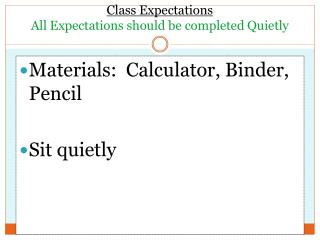# Class Expectations All Expectations should be completed Quietly - PowerPoint PPT PresentationDownload PresentationClass Expectations All Expectations should be completed Quietly

Class Expectations All Expectations should be completed Quietly
Download Presentation## Class Expectations All Expectations should be completed Quietly

- - - - - - - - - - - - - - - - - - - - - - - - - - - E N D - - - - - - - - - - - - - - - - - - - - - - - - - - -
##### Presentation Transcript

1. Class ExpectationsAll Expectations should be completed Quietly Materials: Calculator, Binder, Pencil Sit quietly

2. Ground Works A unit of mathematical riddles

3. Place It Right Goal: students will be able to construct equivalent fractions by using logical reasoning to solve problems.

4. Put one number in each square so that the fractions are equivalent. Use all the numbers given. Number Choices: 1, 0, 2, 4, 3, 5, 6, 8 Place It Right #1

5. Put one number in each square so that the fractions are equivalent. Use all the numbers given. Number Choices: 1, 0, 2, 4, 3, 5, 6, 8 Place It Right #1

6. Put one number in each square so that the fractions are equivalent. Use all the numbers given. Number Choices: 1, 2, 3, 4, 6, 9 Place It Right #2

7. Put one number in each square so that the fractions are equivalent. Use all the numbers given. Number Choices: 1, 2, 3, 4, 6, 9 Place It Right #2

8. Put one number in each square so that the fractions are equivalent. Use all the numbers given. Number Choices: 1, 0, 2, 5, 6, 8, 4 Place It Right #3

9. Put one number in each square so that the fractions are equivalent. Use all the numbers given. Number Choices: 1, 0, 2, 5, 6, 8, 4 Place It Right #3

10. Put one number in each square so that the fractions are equivalent. Use all the numbers given. Number Choices: 1, 0, 2, 7, 4, 3, 5 Place It Right #4

11. Put one number in each square so that the fractions are equivalent. Use all the numbers given. Number Choices: 1, 0, 2, 7, 4, 3, 5 Place It Right #4

12. Put one number in each square so that the fractions are equivalent. Use all the numbers given. Number Choices: 1, 3, 2, 4, 7, 9, 5 Place It Right #5

13. Put one number in each square so that the fractions are equivalent. Use all the numbers given. Number Choices: 1, 3, 2, 4, 7, 9, 5 Place It Right #5

14. Put one number in each square so that the fractions are equivalent. Use all the numbers given. Number Choices: 2, 1, 2, 4, 8, 3, 6, 5 Place It Right #6

15. Put one number in each square so that the fractions are equivalent. Use all the numbers given. Number Choices: 2, 1, 2, 4, 8, 3, 6, 5 Place It Right #6